Note: This is a working paper which will be expanded/updated frequently. All suggestions for improvement are welcome. The directory deleeuwpdx.net/pubfolders/updown has a pdf version, the complete Rmd file with all code chunks, the bib file, and the R source code.

# 1 Introduction

In this paper we study the minimization of stress, defined as $\mathbf{stress}(X):=\frac14\sum_{i=1}^n\sum_{j=1}^n w_{ij}(\delta_{ij}-d_{ij}(X))^2$ over $$n\times p$$ configuration matrices $$X$$. Here $$W=\{w_{ij}\}$$ and $$\Delta=\{\delta_{ij}\}$$ are given symmetric, hollow, and non-negative matrices of, respectively, weights and dissimilarities. The $$d_{ij}(X)$$ are Euclidean distances between the points with coordinates in the rows of $$X$$. Thus $d_{ij}^2(X):=(x_i-x_j)'(x_i-x_j)=(e_i-e_j)'XX'(e_i-e_j)=\mathbf{tr}\ X'A_{ij}X.$ Here $$e_i$$ and $$e_j$$ are unit vectors, with all elements equal to zero except for the single element $$i$$ or $$j$$, which is equal to one. Also $$A_{ij}:=(e_i-e_j)(e_i-e_j)'$$.

The problem of minimizing stress is well-studied (see, for example, Borg and Groenen (2005)). In this paper we analyze the problem of minimizing stress over all configurations $$X$$ for which $$\alpha_{ij}\leq d_{ij}(X)\leq\beta_{ij}$$ for some or all pairs $$i,j$$, where the $$\alpha_{ij}$$ and $$\beta_{ij}$$ are given bounds satisfying $$0\leq\alpha_{ij}<\beta_{ij}\leq+\infty$$. We suppose the constraints are strongly consistent, i.e. there is at least one $$n\times p$$ configuration matrix $$X$$ such that $$\alpha_{ij}<d_{ij}(X)<\beta_{ij}$$.

These optimization problems are non-standard in various ways. Although the set of $$X$$ such that $$d_{ij}(X)\leq\beta_{ij}$$ is convex, the constraints $$d_{ij}(X)\geq\alpha_{ij}$$ define a reverse convex set (Meyer (1970)) in configuration space. The loss funcion stress is neither convex nor concave. We can, however, use basic smacof theory (De Leeuw (1977)) to majorize stress by a convex quadratic and to majorize the constraints by linear or convex quadratic functions. Majorization can be used to define a simple iterative algorithm. In each iteration we majorize stress and the constraints, and then minimize the convex quadratic majorizer over configurations satisfying the majorized constraints using quadratic programming with quadratic constraints or QPQC (De Leeuw (2017c)). Compute a new majorization at the minimizer of the majorized problem, and so on. These are the two steps in the majorization or MM approach to optimization (De Leeuw (1994), Lange (2016)).

## 1.1 Simplifying stress

A simplified expression for stress, first used in De Leeuw (1993), is $\mathbf{stress}(x)=\frac12\sum_{k=1}^K w_k(\delta_k-\sqrt{x'A_kx})^2.$ We have put the elements below the diagonal of $$W$$ and $$\Delta$$ in a vector of length $$\frac12 n(n-1)$$. Also $$x:=\mathbf{vec}(X)$$ and the $$A_k$$ are the direct sums of $$p$$ copies of the corresponding $$A_{ij}$$. Now expand the square, and assume without loss of generality that $$\frac12\sum_{k=1}^K w_k\delta_k^2=1$$. Then $\mathbf{stress}(x)=1-\sum_{k=1}^K w_k\delta_k\sqrt{x'A_kx}+\frac12 x'Vx,$ with $$V:=\sum_{k=1}^K w_kA_k$$.

Suppose $$V=K\Lambda^2K'$$ is a complete eigen-decomposition of $$V$$. Some care is needed to handle the fact that $$V$$ is singular, but under the assumption of irreducibility (De Leeuw (1977)) this is easily taken care of. Change variables with $$z=\Lambda^{\frac12}K'x$$. Then $\mathbf{stress}(z)=1-\sum_{k=1}^K w_k\delta_k\sqrt{z'\tilde A_kz}+\frac12 z'z,$ with $$\tilde A_k=\Lambda^{-\frac12}K'A_kK\Lambda^{-\frac12}$$, so that $$\sum_{k=1}^K w_k\tilde A_k=I$$.

## 1.2 Smacof Notation and Theory

We have see in the previous section that we can write $\mathbf{stress}(x)=1-\rho(x)+\frac12 x'x,$ where $\rho(x):=\sum_{k=1}^K w_k\delta_k d_k(x),$ with distances $$d_k(x):=\sqrt{x'A_kx}$$, and the $$A_k$$ positive semi-definite matrices that add up to the identity. By Cauchy-Schwarz, $\rho(x)=x'B(x)x\geq x'B(y)y,$ where $B(x):=\sum_{k=1}^K w_k\frac{\delta_k}{d_k(x)}A_k.$ If we define the Guttman transform if $$x$$ as $$\Gamma(x):=B(x)x$$, then for all $$x$$ $\mathbf(stress)(x)=1-x'\Gamma(x)+\frac12 x'x=(1-\frac12\Gamma(x)'\Gamma(x))+\frac12(x-\Gamma(x))'(x-\Gamma(x)),$ and for all $$x,y$$ $\mathbf(stress)\leq 1-x'\Gamma(y)+\frac12 x'x=(1-\frac12\Gamma(y)'\Gamma(y))+\frac12(x-\Gamma(y)'(x-\Gamma(y)),$ In this notation a smacof iteration is $$x^{(k+1)}=\Gamma(x^{(k)})$$.

# 2 Some Majorization Theory

Suppose the problem we are interested in is to minimize a real-valued $$f_0$$ over all $$x\in\mathbb{R}^n$$ that satisfy $$f_j(x)\leq 0$$ for all $$j=1,\cdots,m$$. We call this problem $$\mathcal{P}$$.

Now suppose $$g_j:\mathbb{R}^n\otimes\mathbb{R}^n\rightarrow\mathbb{R}$$ majorizes $$f_j$$, i.e. \begin{align*} f_j(x)&\leq g_j(x,y)\text{ for all }x,y\in\mathbb{R}^n,\\ f_j(y)&=g_j(y,y)\text{ for all }y\in\mathbb{R}^n. \end{align*}

To simplify matters we suppose all $$f_j$$ and $$g_j$$ are continuously differentiable, and all $$g_j$$ are convex in their first argument. Define problem $$\mathcal{P}_k$$ as the problem of minimizing $$g_0(x,x^{(k-1)})$$ over the $$x\in\mathbb{R}^n$$ that satisfy $$g_j(x,x^{(k-1)})\leq 0$$. Define a sequence $$x^{(k)}$$ with $$x^{(0)}$$ feasible for $$\mathcal{P}$$, and with $$x^{(k)}$$ for $$k\geq 1$$ a solution of $$\mathcal{P}_k$$.

Theorem 1: [Convergence]

1. $$x^{(k)}$$ is feasible for $$\mathcal{P}$$ for all $$k\geq 1$$.
2. $$f_0(x^{(k)})\leq f_0(x^{(k-1)})$$ for all $$k\geq 1$$.

Proof: For the first part $$f_j(x^{(k)})\leq g_j(x^{(k)},x^{(k-1)})$$ by majorization, and $$g_j(x^{(k)},x^{(k-1)})\leq 0$$ by feasibility for $$\mathcal{P}_k$$. For the second part $$f_0(x^{(k)})\leq g_0(x^{(k)},x^{(k-1)})$$ by majorization. Because $$x^{(k)}$$ solves $$\mathcal{P}_k$$ we have $$g_0(x^{(k)},x^{(k-1)})\leq g_0(x^{(k-1)},x^{(k-1)})$$ if $$x^{(k-1)}$$ is feasible for $$\mathcal{P}_k$$, i.e. if $$g_j(x^{(k-1)},x^{(k-1)})\leq 0$$. But $$g_j(x^{(k-1)},x^{(k-1)})=f_j(x^{(k-1)})\leq 0$$ by the first part of the theorem. QED

## 2.1 Fixed Points

By theorem 1 we have a sequence of points with decreasing function values that are all feasible for $$\mathcal{P}$$. We can say a bit more about accumulation points of this sequence. Define $$\mathcal{P}(y)$$ as the convex problem of minimizing $$g_0(x,y)$$ over $$x\in\mathbb{R}^n$$ and $$g_j(x,y)\leq 0$$.

Theorem 2: [Necessary]

If $$x$$ solves $$\mathcal{P}(x)$$ then $$x$$ satisfies the first order necessary conditions for a local minimum of $$\mathcal{P}$$.

Proof: The necessary and sufficient conditions for $$x$$ to be a minimum of $$\mathcal{P}(y)$$ are that there exists $$\lambda\geq 0$$ such that $\mathcal{D}_1g_0(x,y)+\sum_{j=1}^m\lambda_j\mathcal{D}_1g_j(x,y)=0,$ with in addition $$g_j(x,y)\leq 0$$ and $$\lambda_jg_j(x,y)=0$$.

The first order necessary conditions for $$x$$ to be a local minimum of $$\mathcal{P}$$ are that there exists $$\lambda\geq 0$$ such that $\mathcal{D}f_0(x)+\sum_{j=1}^m\lambda_j\mathcal{D}f_j(x)=0,$ with in addition $$f_j(x)\leq 0$$ and $$\lambda_jf_j(x)=0$$.

But if the $$f_j$$ and $$g_j$$ are differentiable, we know from majorization theory (De Leeuw (2016), Lange (2016)) that $$\mathcal{D}f_j(x)=\mathcal{D}_1g_j(x,x)$$ for all $$x$$.

QED

It follows that if we define $$\mathcal{F}(y)$$ as the solution of $$\mathcal{P}(y)$$ then fixed points of $$\mathcal{F}$$ are local minimizers of $$\mathcal{P}$$. Thus if $$x^{(k)}$$ converges to a fixed point of $$\mathcal{F}$$, it converges to a local minimizer of $$\mathcal{P}$$, and $$f_0(x^{(k)})$$ converges to a local minimum.

# 3 MDS with Distance Bounds

There are two types of constraints. The first are $$\sqrt{x'A_kx}\leq\beta_k$$, which we transform simply to $\begin{equation}\label{E:up} x'A_kx\leq\beta_k^2 \end{equation}$

and otherwise leave undisturbed. No majorization is required.

The second set of constraints is $$\sqrt{x'A_kx}\geq\alpha_k$$ or $$\alpha_k-\sqrt{x'A_kx}\leq 0$$. We majorize at $$x^{(k-1)}$$, using Cauchy-Schwarz, to $\begin{equation}\label{E:down} \alpha_k-\frac{1}{d_k(x^{(k-1)})}x'A_kx^{(k-1)}\leq 0 \end{equation}$

Thus the QPQC problem we have to solve in each bounded smacof iteration, a.k.a. problem $$\mathcal{P}_k$$, is minimization of the quadratic $$1-x'\Gamma(x^{(k-1)})+\frac12 x'x$$ over $$x$$ satisfying the quadratic constraints $$\eqref{E:up}$$ and the linear constraints $$\eqref{E:down}$$.

# 4 Software

Minimizing a convex quadratic under convex quadratic constraints has been implemented in the R function qpqc, described in De Leeuw (2017c). It dualizes the problem and then uses the nnnewtin function for Newton’s method with non-negativity constraints. We apply qpqc to solve our problems $$\mathcal{P}_k$$, using the fact that the linear constraints $$\eqref{E:down}$$ are just quadratic constraints with a zero quadratic component.

The smacofUpDown function has two arguments without default values, a vector delta of length $$\frac12n(n-1)$$ with dissimilarities (lower-diagonal, columnwise, same ordering as a dist object) and an $$n\times p$$ matrix xini with an initial configuration.

args(smacofUpDown)
## function (delta, xini, w = rep(1, length(delta)), bndlw = rep(0,
##     length(delta)), bndup = rep(Inf, length(delta)), itmax = 1000,
##     eps = 1e-10, verbose = FALSE)
## NULL

The arguments bndlw and bndup are vectors of length $$\frac12n(n-1)$$, with default values 0 and $$+\infty$$. Thus, by default, smacofUpDown computes an unconstrained weighted least squares multidimensional scaling solution, where the weights in w are by default all equal to $$+1$$.

There is no default value for xini, and the user must make sure that the distances of the initial configuration are between the bounds (are feasible). This is easy enough to do if there are only lower bounds (take any configuration and make it big enough) or only uppper bounds (make it small enough). If there are both upper and lower bounds then feasibility should be checked, because smacofUpDown will refuse to start if the initial configuration has any infeasible distances.

# 5 Examples

## 5.1 Equidistances

Our first example has $$n=10$$ with all $$\delta_{ij}$$ equal. The optimal smacof solution in two dimensions needs 131 iterations to arrive at stress 0.1098799783. We reanalyze the data with the constraint that the distance between the first point and all nine others is at least one. The algorithm incorporating these bounds, implemented in the function smacofAbove, uses 156 iterations and finds stress 0.1340105193. The two configurations are in figure 1. There are five active constraints, i.e. distances equal to one, indicated by lines in the plot.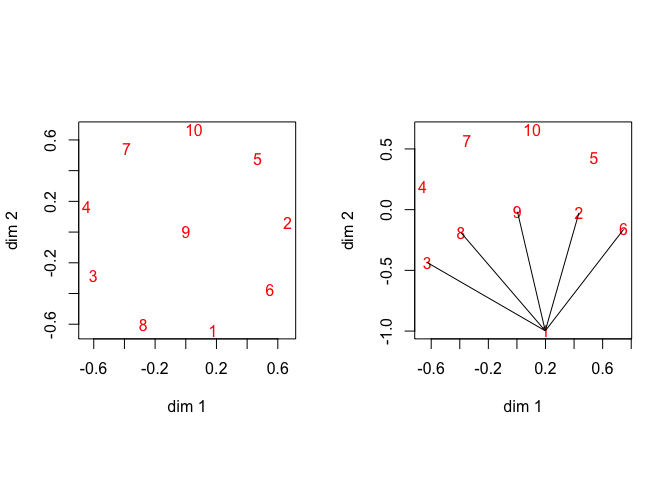Figure 1: Equidistance Data, Unconstrained Left, Lower Bounds Right

In the second analysis, now using eight objects with equal dissimilarties, we require $$d_{ij}(X)\geq 1$$ for all $$i,j$$ with $$|i-j|=1$$, and $$d_{ij}(X)\leq 2$$ for all $$i,j$$ with $$|i-j|=2$$.

In the unconstrained case we find stress equal to 0.0973520592 after 132 iterations, in the constrained case we find 0.1183956861 after 357 iterations. The lower bound constraints are all active, which means the distances between successive points are all one (indicated with lines in figure 2. The upper bound constraints are all inactive.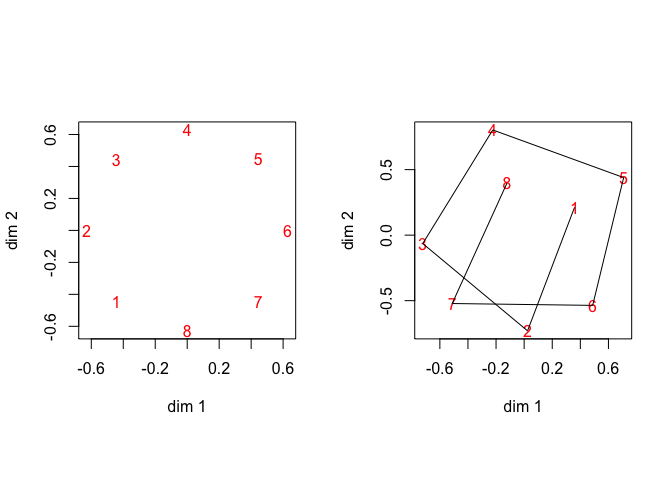Figure 2: Equidistance Data, Unconstrained Left, Lower Bounds Right

So far we have assumed $$\alpha_{ij}<\beta_{ij}$$ for all $$i,j$$. It is possible, however, to let $$\alpha_{ij}=\beta_{ij}$$, which means we require $$d_{ij}(X)=\alpha_{ij}=\beta_{ij}$$. Note the complete set of constraints still needs to be consistent, and the initial configuration must still be feasible. In our example we require all seven distances between successive points to be equal to one.

Our algorithm in this case becomes extremely slow. It stops at the upper bound of 2000 iterations, with stress 0.145422896. Of course all constraints are now active. The Lagrange multipliers are large, serving as penalty parameters to force the equality constraints.

##   607.209037 798.640263 184.410120 470.471412 837.258144 684.253342
##   342.484954 607.726977 799.263569 184.916274 470.878355 838.697106
##  685.017712 342.476621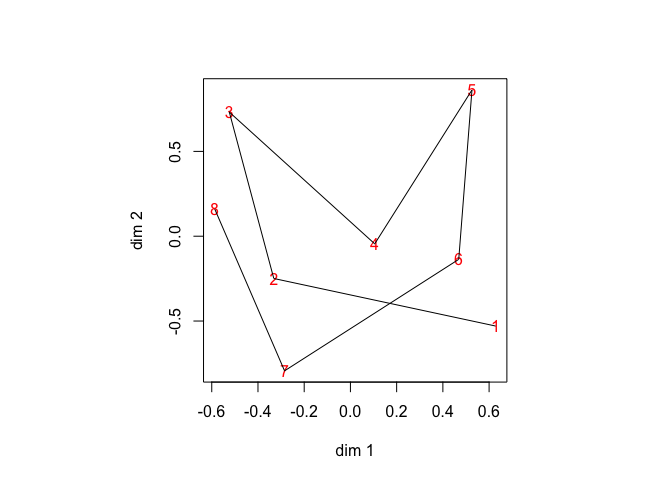Figure 3: Equidistance Data, Distances Constrained to One

Note, however, that the previous analysis where we merly required $$d_{ij}(X)\geq 1$$ for $$|i-j|=1$$ produced a solution feasible for the current problem with stress equal to 0.1183956861. This makes it interesting see what goes on if we increase the upper bound of the number of iterations even more, say, to 10000.

We now find stress 0.1016650356 after 4638 iterations. The Lagrange multipliers for this solution are much smaller (first seven correspond with upper bounds, second seven with lower bounds). What basically happens is that for each $$(i,j)$$ with $$|i-j|=1$$ either the upper or the lower constraint is seen to be violated and gets a zero Lagrange multiplier. The corresponding Lagrange multiplier for the corresponding other constraint is positive, because it is interpreted as being satisfied. This is a consequence of using floating point with limited precision, and the difference between $$\alpha_{ij}=0.99\cdots$$ and $$\beta_{ij}=1.00\cdots$$.

##  0.1769885825 0.0000000000 0.2256740368 0.0000000001 0.0000005800
##  0.0000000012 0.0707712910
##  0.0000094723 0.2413717195 0.0000020656 0.0233523251 0.1287322429
##  0.1247717131 0.0000000002

The successive distances are

##  0.9999999397 1.0000000032 1.0000000368 1.0000000221 0.9999999739
##  0.9999999647 0.9999999492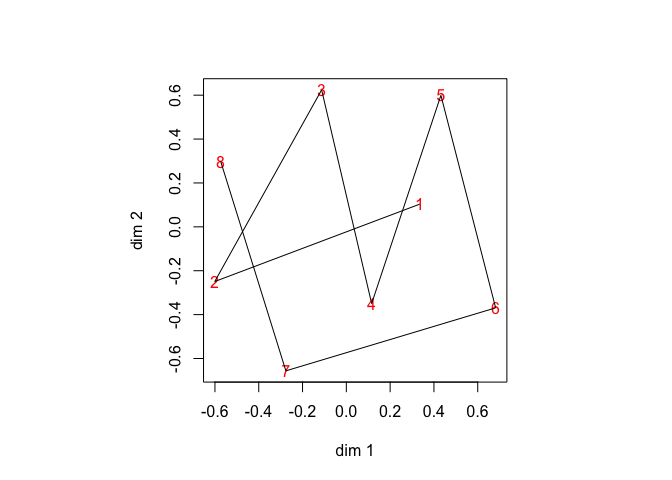Figure 4: Equidistance Data, Distances Constrained to One

## 5.2 Dutch Political Parties 1967

As the next illustration we use data from De Gruijter (1967), with average dissimilarity judgments between Dutch political parties in 1967. The data are

##       KVP PvdA  VVD  ARP  CHU  CPN  PSP   BP
## PvdA 5.63
## VVD  5.27 6.72
## ARP  4.60 5.64 5.46
## CHU  4.80 6.22 4.97 3.20
## CPN  7.54 5.12 8.13 7.84 7.80
## PSP  6.73 4.59 7.55 6.73 7.08 4.08
## BP   7.18 7.22 6.90 7.28 6.96 6.34 6.88
## D66  6.17 5.47 4.67 6.13 6.04 7.42 6.36 7.36

First, three different but comparable anlyses are done. The first does not impose restriction, the second is MDS from below, which requires $$d_{ij}(X)\leq\delta_{ij}$$ for all $$i,j$$. And the third is MDS from above, for which $$d_{ij}(X)\geq\delta_{ij}$$ for all $$i,j$$. The configurations are quite similar, except for the position of D’66, which at the time was a novelty in Dutch politics. The value of stress at the solutions is, respectively, 0.044603386, 0.0752702106, and 0.280130691.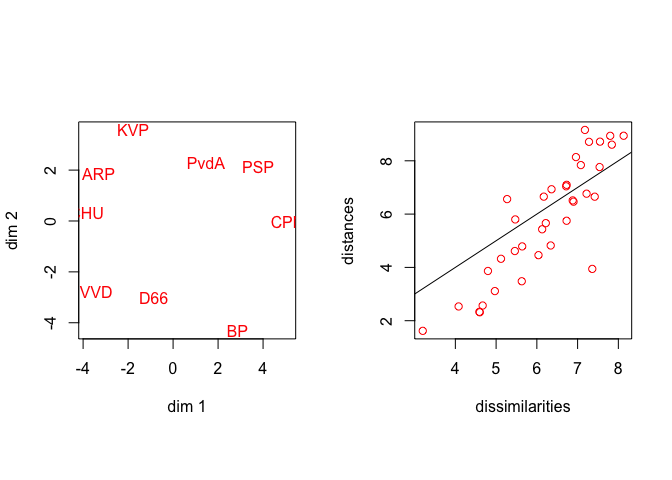Figure 5: De Gruijter Data, Unconstrained Solution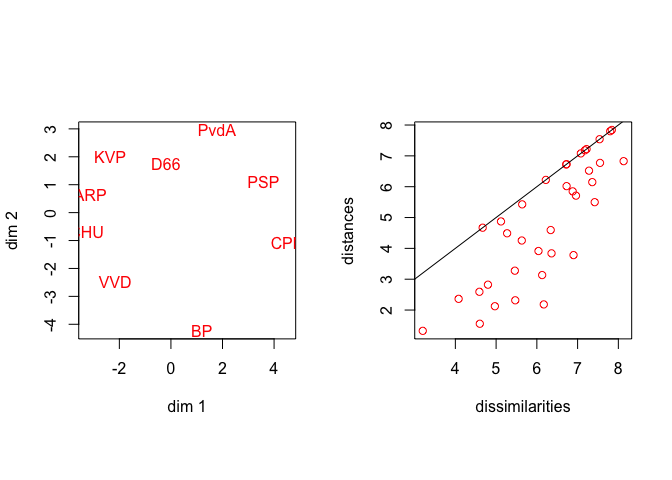Figure 6: De Gruijter Data, MDS from Below